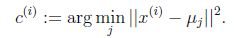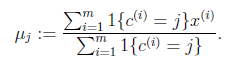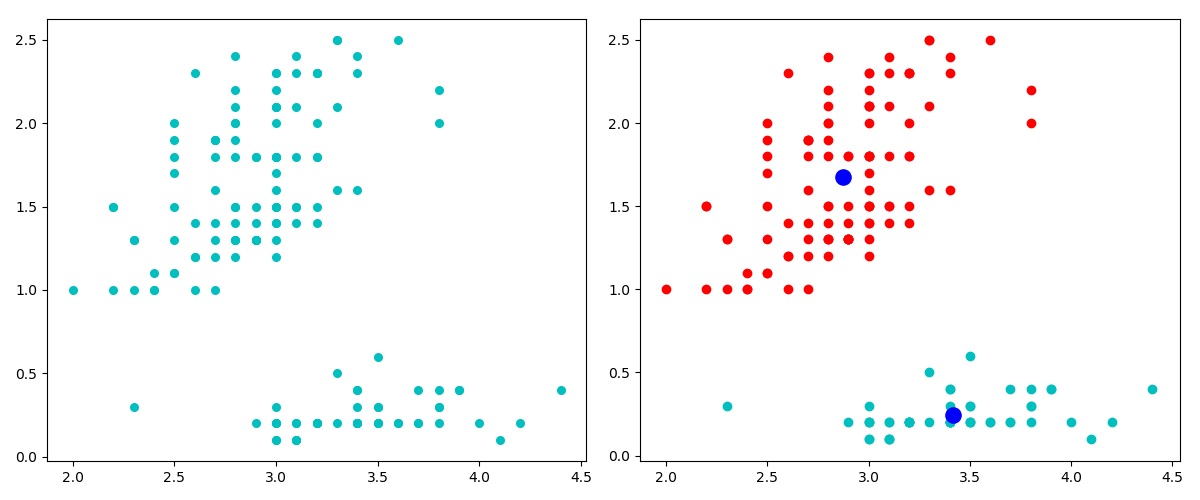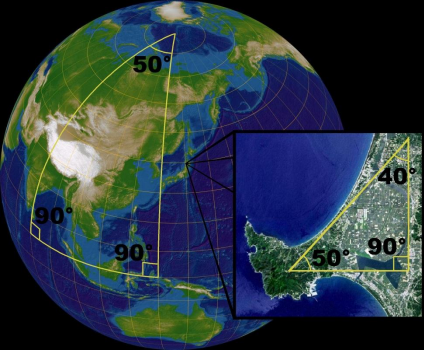# K-means## 标准算法

• 分配(Assignment)：将每个样本i分配到聚类中，使得组内平方和（WCSS）达到最小。•  更新(Update)：对于上一步得到的每一个聚类，以聚类中样本值的图心，作为新的均值点。K-means面对的第一个问题是如何保证收敛，首先可以固定每个类的质心 ，调整每个样本的所属类别  来让WCSS减小；同样固定  ，调整每个类的质心  也可以让WCSS减小，这两个过程就是内循环中使J单调递减的过程。当WCSS递减到最小时，和c也同时收敛。（在理论上，可以有多组不同的和c值能够使得J取得最小值，但这种现象实际上很少见）。

## iris

from sklearn import datasets
from matplotlib import pyplot as plt
import numpy as np
X, y = iris.data, iris.target
data = X[:,[1,3]] # 为了便于可视化，只取两个维度
fig, plt.scatter(data[:,0],data[:,1])
plt.show()

## 欧式距离

def distance(p1,p2):
tmp = np.sum((p1-p2)**2)
return np.sqrt(tmp)

## 随机质心

def rand_center(data, k):
"""Generate k center within the range of data set."""
n = data.shape
centroids = np.zeros((k,n)) # k个质心，每个质心的维度和样本维度相同
for i in range(n):
dmin, dmax = np.min(data[:, i]), np.max(data[:, i])
centroids[:, i] = dmin + (dmax - dmin) * np.random.rand(k)
return centroids

centroids = rand_center(data,2)
centroids
array([[ 2.15198267,  2.42476808],
[ 2.77985426,  0.57839675]])

## 完整代码

kmeans有个缺点，就是可能陷入局部最小值，有改进的方法，比如二分k均值；也可以多计算几次，取效果好的结果。

def kmeans(data,k=2):
def _distance(p1,p2):
tmp = np.sum((p1-p2)**2)
return np.sqrt(tmp)

def _rand_center(data,k):
"""Generate k center within the range of data set."""
n = data.shape # features
centroids = np.zeros((k,n))
for i in range(n):
dmin, dmax = np.min(data[:,i]), np.max(data[:,i])
centroids[:,i] = dmin + (dmax - dmin) * np.random.rand(k)
return centroids

def _converged(centroids1, centroids2):
# if centroids not changed, we say 'converged'
set1 = set([tuple(c) for c in centroids1])
set2 = set([tuple(c) for c in centroids2])
return (set1 == set2)

m = data.shape # number of entries
centroids = _rand_center(data,k)
c = np.zeros(m, dtype=np.int) # track the nearest centroid
assement = np.zeros(m) # for the assement of our model
converged = False

while not converged:
old_centroids = np.copy(centroids)
for i in range(m):
# determine the nearest centroid and track it with label
min_dist, min_index = np.inf, -1
for j in range(k):
dist = _distance(data[i],centroids[j])
if dist < min_dist:
min_dist, min_index = dist, j
c[i] = j
assement[i] = _distance(data[i], centroids[c[i]])**2

# update centroid
for j in range(k):
centroids[j] = np.mean(data[c==j], axis=0)
converged = _converged(old_centroids, centroids)

return centroids, c, np.sum(assement)

best_assement = np.inf
best_centroids = None
best_c = None

for i in range(10):
centroids, c, assement = kmeans(data,2)
if assement < best_assement:
best_assement = assement
best_centroids = centroids
best_c = c

data0 = data[best_c==0]
data1 = data[best_c==1]

fig, (ax1,ax2) = plt.subplots(1,2,figsize=(12,5))
ax1.scatter(data[:,0],data[:,1],c='c',s=30,marker='o')
ax2.scatter(data0[:,0],data0[:,1],c='r')
ax2.scatter(data1[:,0],data1[:,1],c='c')
ax2.scatter(centroids[:,0],centroids[:,1],c='b',s=120,marker='o')
plt.show()# 最大似然估计

## 为什么叫「最大似然（最大可能性）」，而不是「最大概率」呢？# Kullback–Leibler divergence

KL散度是两个概率分布P和Q差别的非对称性的度量。 KL散度是用来 度量使用基于Q的编码来编码来自P的样本平均所需的额外的位元数。 典型情况下，P表示数据的真实分布，Q表示数据的理论分布，模型分布，或P的近似分布。

## 定义对于连续随机变量，其概率分布P和Q可按积分方式定义为# 流形（manifolds）

• 不满足平行公设：存在过空间中任意两点的平行直线(测地线)• 球面：任意两条测地线(大圆弧)都相交
• 测地三角形的内角和不一定等于180度

12-234万+

#### 计算机视觉如何入门

05-182万+

#### 计算机视觉入门01-105359

#### 基于灰度图像的质心算法和三角测距原理（学习笔记）

04-023万+

#### Fuzzy C-Means（模糊C均值聚类）算法原理详解与python实现

12-221万+

#### 如何学习计算机视觉

11-241171

#### 数据学习(5)·K-means 聚类和PCA算法

06-138354

#### 算法导论习题解-第15章动态规划

05-293166

#### 机器学习（九）-k-means算法及优化和Python

12-28422

#### 裹动智驾自动驾驶测试工程师笔试题面试题

04-253878

#### java实现k-means算法（用的鸢尾花iris的数据集，从mysq数据库中读取数据）©️2020 CSDN 皮肤主题: 大白 设计师: CSDN官方博客点击重新获取扫码支付1.余额是钱包充值的虚拟货币，按照1:1的比例进行支付金额的抵扣。
2.余额无法直接购买下载，可以购买VIP、C币套餐、付费专栏及课程。余额充值